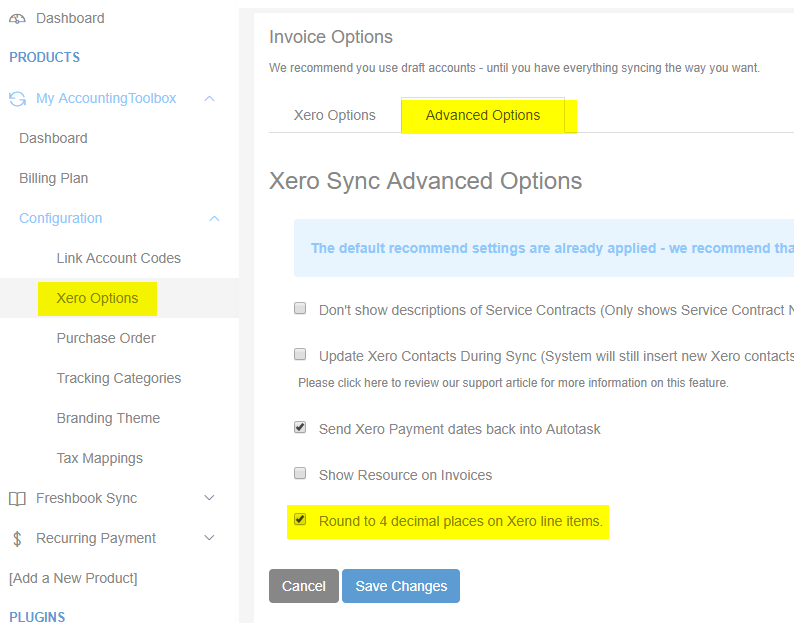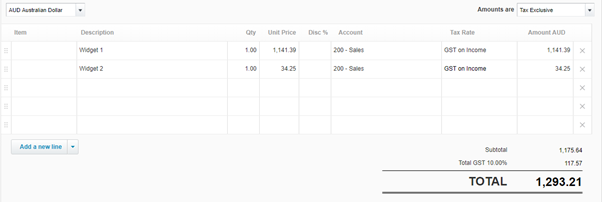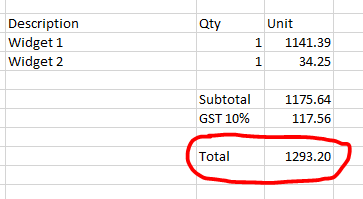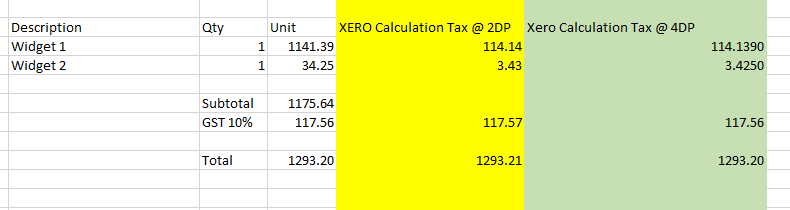# Rounding issues in Xero invoices

## Autotask and Xero now supports four decimal places, you can activate four decimal places, under advanced settings in Xero Options in our console.

Xero and Autotask use slightly different methodologies to calculate tax rates this can lead to minor rounding differences between the two platforms.

Turning on 4 decimal place rounding in Autotask and Cloud Depot can reduce the rounding variances between the two platforms.Please note four decimal place support needs to be activated in Autotask, no change is required in Xero.

### Here is an explanation of how Xero calculates tax amounts and why it can sometimes differ from other platforms.

In Xero, taxes are calculated individually for each line-item. By default, each line-item is rounded to two decimal places. These rounded amounts are then added together to determine the overall taxable amount. This methodology can have an impact on the final result, as demonstrated in the example below.

Let's consider an example of two products being sold in Xero with a tax rate of 10%.

• widget 1 for 1141.39
• widget 2 for 34.25Xero calculates the GST @ 10% to be \$117.57

If you try to apply the same calculation in Microsoft Excel, you may notice that the total is off by 1 cent compared to the example in Xero.This discrepancy occurs because the tax calculation is done on each individual line-item and then rounded to two decimal places, resulting in a variation in the overall calculation. If your invoice contains multiple line items, this rounding difference will be more pronounced.